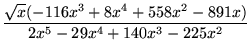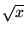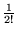Next: If the Curve Fits, Up: Preliminaries about Maple Previous: Plotting with Maple

# Exercises

1. Evaluateto 30 decimal places.
2. Input the following as a Maple function:

f (x) =.

a)
Write this in a simpler form (that is, factor and reduce it).
b)
Draw the graph of the function for x between 0 and 1). Adjust the vertical range so that some detail can be seen.
c)
Compute the area of the part of the curve that lies above the x-axis and between x = 0 and x = 5 (that is, integrate the function over the appropriate range of x values). Give your answer both in actual form and as a decimal approximation to about 10 places.
d)
What is the value of the integral if you remove the factor offrom the numerator?
e)
Use the derivative of f (x) to determine for what real value x the function has a local maximum. Use an approximation to about 8 decimal places.
3. Use the commands seq and ithprime to generate a list of the first 20 primes. Compute the sum of these 20 primes, and give its integer factors.
4. Find the solutions of the system of equations {x2 - y2 = 4, x - 2y = 2}.
5. Draw a graph showing both cosx and its fifth Taylor polynomial (that is, 1 -x2 +x4) for x between -4 and 4. How many terms do you seem to need to get good agreement in this range.

Hint: use a variation of the command convert(taylor(cos(x),x,5),polynom) to make this work.

Think of a suitable way to demonstrate that the approximation you have taken is good.Next: If the Curve Fits, Up: Preliminaries about Maple Previous: Plotting with Maple

Translated from LaTeX by Scott Sutherland
2002-08-29